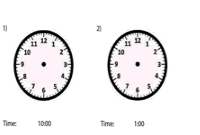# What Time Is It? Worksheets

How to Draw the Hands on a Clock to Represent Time - Making your children learn about time is a hectic task, given that a normal analog clock doesn’t’ have much information to begin with. On the other hand, you cannot rely on a digital clock to make understanding easier. Thus, this is where drawing hands to represent time is a necessary learning objective. Now, the basic rule of math is practice makes perfect, which is exactly what is required here. In other words, for your students to learn the clock representation, they must practice drawing hands on a clock regularly. The key to practice is you must devise questions by performing increment of different durations. Let us consider an example:Now, the students must select a time on the left clock by drawing hands. And then, they can increment an hour to the original time. So, if the first clock shows half past 9, then the next one will show at half-past 10.

• ### Basic Lesson

Students learn the significance of the minute and hour hands on clocks. Includes 2 practice problems. The duration of a day is split into 24 hours and each of those hours is split into 60 minutes. In a clock, each hour is subdivided with a gap of 5 minutes i.e. 5, 10, 15, 20, 25………. If the minute hand is on 1 it corresponds to 5 minutes, on 2 it corresponds to 10 minutes, and so on. If the small hand (hour hand) is between 2 numbers, the lower number is the hour, e.g. hour hand is between 5 and 6, and the minute hand is on 30 minutes, the time is read as 5:30. This is not the case when the hour hand is between 12 and 1; Then the hour is 11, e.g the minute hand is on the 15 minutes, it is 12:15.

• ### Intermediate Lesson

Practice drawing the minute and hour hand on clocks.

• ### Independent Practice 1

Practice telling time and writing the numbers and words for time. For each of the clocks, write the time.

• ### Independent Practice 2

Students draw the minute and hour hands on clocks.

• ### Homework Worksheet

Walks students through both telling time and drawing hands on the clocks.

• ### Skill Quiz

6 problems ask students to tell time. 4 problems ask students to draw the hands on the clocks.

• ### Homework and Quiz Answer Key

Answers for the homework and quiz.